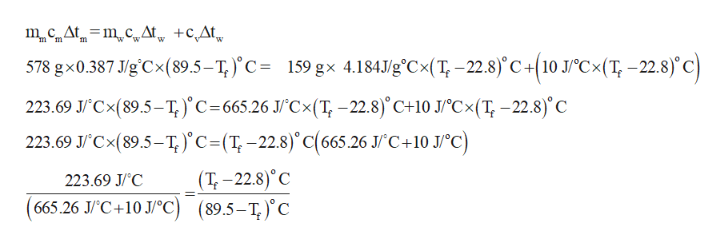# please help i have no ideaA 578−g piece of copper tubing is heated to 89.5°C and placed in an insulated vessel containing 159 g of water at 22.8°C. Assuming no loss of water and a heat capacity for the vessel of 10.0 J/°C, what is the final temperature of the system (c of copper = 0.387 J/g·°C)?

Question
34 views

A 578−g piece of copper tubing is heated to 89.5°C and placed in an insulated vessel containing 159 g of water at 22.8°C. Assuming no loss of water and a heat capacity for the vessel of 10.0 J/°C, what is the final temperature of the system (c of copper = 0.387 J/g·°C)?

check_circle

Step 1

The amount of heat lost by the metal(qm) is equal to amount of heat gained by water(qw) and vessel qv . This is shown in equation (1) in which mm is the mass of metal copper, cm is the specific heat for metal, ∆tm is the change in temperature of the metal, mw is the mass of water , cw is the specific heat for water,  ∆tis the change in temperature of the water and cv is the heat capacity for vessel.

Step 2

Substitute 578 g for mm, (89.5- Tf)○C for ∆tm, 0.387 J/g.○C 159 g for mw, 4.184 J/g○C for cw and (Tf -22.8)○C for ∆tw  and 10.0 J/○C for c...help_outlineImage TranscriptionclosemCAtmcAt +cAt w 578 gx0.387 J/g'Cx(89.5-T,)'c= 159 gx 4.184Jg'Cx(T-22.8)'C+10 J°C<(T -22.8)'c 223.69 J'Cx(89.5-T, )'C 665.26 J/Cx(T,-22.8) C+10 J/°Cx(T,-22.8)'C 223.69 JCx(89.5-T,)'C= (T,-22.8)'C(665.26 J/"C +10 J/°C) (T-22.8) C (665.26 J/C+10 J/C) (89.5-T) C 223.69 J/C = fullscreen

### Want to see the full answer?

See Solution

#### Want to see this answer and more?

Solutions are written by subject experts who are available 24/7. Questions are typically answered within 1 hour.*

See Solution
*Response times may vary by subject and question.
Tagged in

### Chemistry